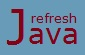# Array and String Programs in Java

In this tutorial we will see some of the programs of array and string which are commonly asked with beginners in interviews. These programs will help them to build their logics in using array and string. Let's see the first program which shows how to check if a given number exist inside an array. If it exist, the program will also print it's index in the array.

### Program to search an element in an array

```` class` SearchNumber
{
`public static void` main(String [] args)
{
`int` [] numbers = {40,60,80,65,70};
`boolean` isExist = `false`;
`int` searchNumber = 80;
`int` pos = -1;
`for`(`int` i = 0; i < numbers.length; i++)
{
`if`(numbers[i] == searchNumber)
{
isExist = `true`;
pos = i;
`break`;
}
}
`if`(isExist)
System.out.println(`"number "`+searchNumber+`" exist in the array at index = "`+pos);
`else`
System.out.println(`"number "`+searchNumber+`" does not exist inside the array"`);
}
}```

Output :

number 80 exist in the array at index = 2

The next program shows how to find minimum and maximum element of an array. This is one way to find the minimum and maximum element, there can be other approaches as well.

### Java program to find maximum and minimum number in an array

```` class` MinMaxOfArray
{
`public static void` main(String [] args)
{
`int` [] numbers = {40,20,80,65,70,90,35,10};
`int` maxValue = numbers;
`int` minValue = numbers;
`for`(`int` i=1;i < numbers.length;i++)
{
`if`(numbers[i] < minValue)
minValue = numbers[i];

`if`(numbers[i] > maxValue)
maxValue = numbers[i];
}
System.out.println(`"Minimum number = "`+minValue+`" and maximum number = "`+maxValue);
}
}```

Output :

Minimum number = 10 and maximum number = 90

The next program shows how to sort an array of integer elements in ascending order. This is one approach to sort an array, there are other approaches as well to do the same.

### Java Program to sort an array

```` class` SortArray
{
`public static void` main(String [] args)
{
`int` [] numbers = {40,20,80,65,70,90,35,10};
`int` length = numbers.length;
`int` temp;
`for`(`int` i = 0; i < length; i++)
{
`for`(`int` j = i + 1; j < length; j++)
{
`if`(numbers[i] > numbers[j])
{
temp = numbers[i];
numbers[i] = numbers[j];
numbers[j] = temp;
}
}
}
System.out.println(`"Array elements in ascending Order:"`);
`for`(`int` n : numbers)
System.out.print(n + `" "`);
}
}```

Output :

Array elements in ascending Order:
10 20 35 40 65 70 80 90

The next program shows how to reverse a given string and checks if it is a palindrome string. A string is called palindrome if the reverse of the string is same as the original string. Again this is one way to reverse the string, there can be other ways as well to do the same. You can also use reverse() method of StringBuffer/StringBuilder class to reverse a given string directly but our intention is to learn the logic.

### Java Program to reverse a string and palindrome string

```` class` ReverseAndPalindromeString
{
`public static void` main(String [] args)
{
String str =  `"abcba"`;
String revStr = `""`;
`for`(`int` i = str.length() - 1; i >= 0; i--)
{
revStr = revStr + str.charAt(i);
}
System.out.println(`"Reverse string = "`+revStr);
`if`(revStr.equals(str))
System.out.println(`"The given string is a palindrome string"`);
`else`
System.out.println(`"The given string is not a palindrome string"`);
}
}```

Output :

Reverse string = abcba
The given string is a palindrome string

Let's write one more program which counts total number of occurrence of a given character inside a given string. The program shows two different approaches to count the total occurrence.

### Program to find occurrence of a character in a string in Java

```` class` CharacterCount
{
`public static void` main(String[] args)
{
String str = `"Java is easy to learn"`;
`char` c = `'a'`;
`int` count = 0;
`// First Approach`
`char`[] charArray = str.toCharArray();
`for`(`char` ch : charArray)
{
`if`(ch == c)
count++;
}
System.out.println(`"Total occurrence of character 'a' using 1st approach = "`+count);

`// Second Approach`
`int` count2 = str.length() - str.replace(`"a"`, `""`).length();
System.out.println(`"Total occurrence of character 'a' using 2nd approach = "`+count2);
}
}```

Output :

Total occurrence of character 'a' using 1st approach = 4
Total occurrence of character 'a' using 2nd approach = 4